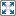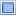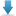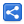# Groovy - Imports

[Updated: Feb 23, 2019, Created: Feb 23, 2019]

Similar to Java, Groovy supports all kind of import statements.

In this tutorial we will go though the additional import features provided by groovy.

## Default Imports

Following default imports are provided by groovy:

```import java.lang.*
import java.util.*
import java.io.*
import java.net.*
import groovy.lang.*
import groovy.util.*
import java.math.BigInteger
import java.math.BigDecimal```

That means we don't have to explicitly use any of above imports.

### Example

```//no need to use import
def list = List.of(1, 3,)
def date = new Date();
def file = new File("c:/temp")

//needs imports for other
import java.time.LocalDateTime
def now = LocalDateTime.now()

```

## Static imports

Groovy supports all Java style static imports but additionally it allows us to define methods with the same name as an imported method as long as they have different types.

### Example

```import static java.lang.Math.pow;

int pow(int base, int power) {
println "local pow called"
return base * power

}

def p = pow(2.1d, 3.1d);//will call Math.pow(double, double)
println p
def p2 = pow(1, 3) // will call local pow(int, int)
println p2

```

#### Output

`9.97423999265871local pow called3`

## Import aliasing

The import with the as keyword can be used for aliasing.

### Example

```import java.time.LocalTime as Time
import static java.lang.Math.pow as power

println Time.now()
println power(3,5)

```

#### Output

`03:05:53.289722300243.0`

## Example Project

Dependencies and Technologies Used:

• Groovy 2.5.6
• JDK 11
 Using imports in GroovySelect AllDownload• groovy-default-imports
• src
• Example1DefaultImport.groovy

 Share